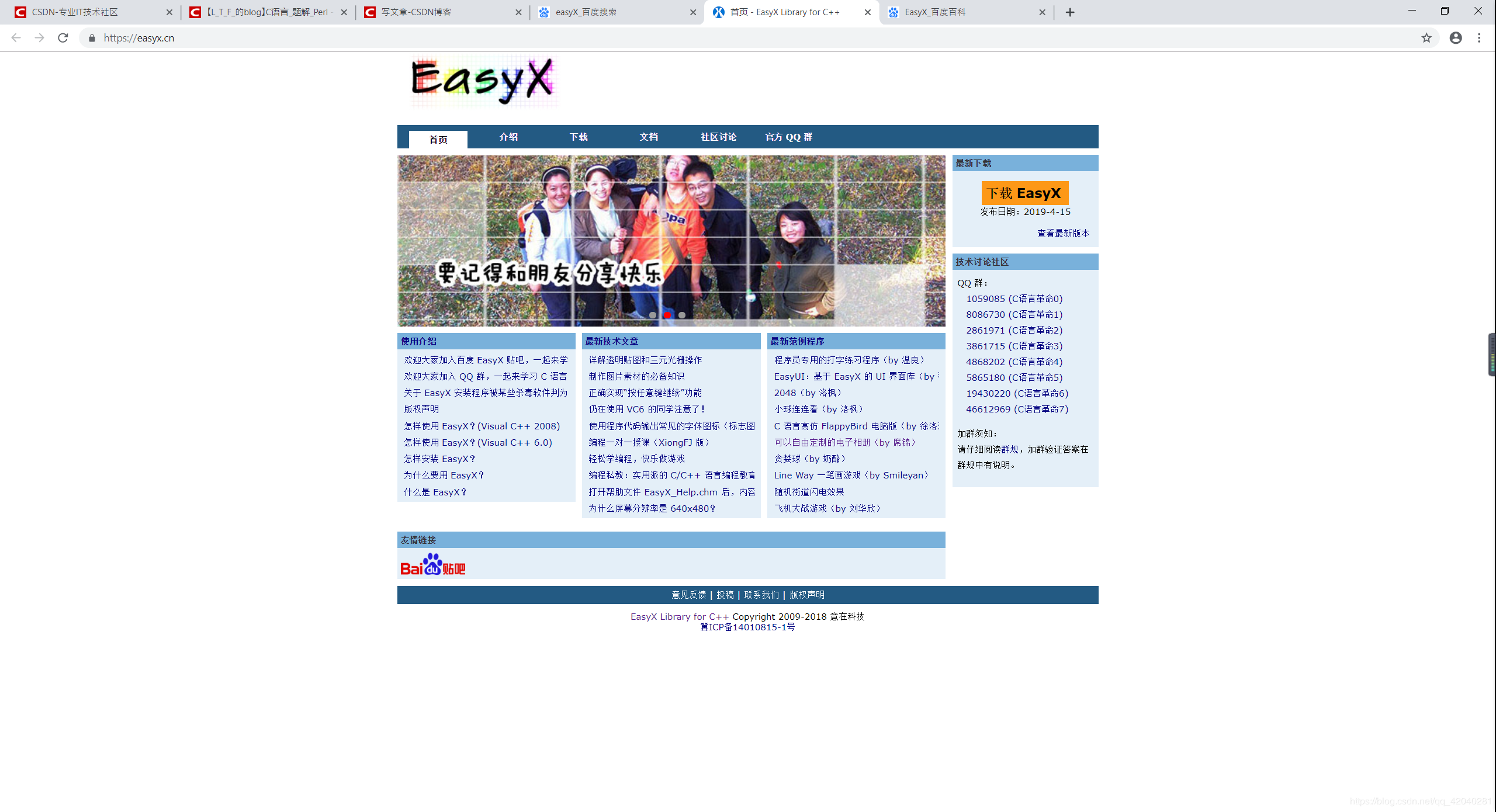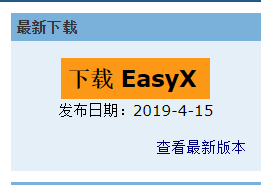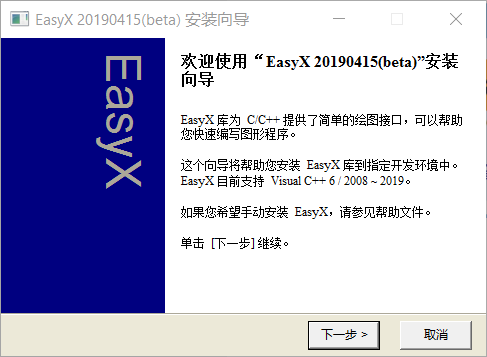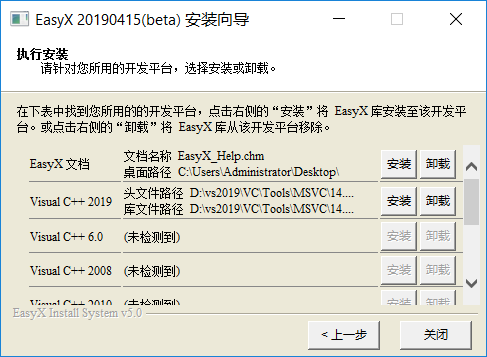Less is More

2019-05-21 13:51:10• 开发个游戏？（我只会控制台）
• 学下人工智能？（脑子不够用）
• 学一下Py？（爱我C++）
• 打文化课？（滚一边去）

• QT？（不会）
• MFC？（算了吧）
• ALT？（这又是啥？）
• Win32？（早过时了）
• EasyX？（这是个什么东西？）

# 关于EasyX

Q:EasyX怎么读？
A:Easy+/eks/或者Easy+叉（

Q:他是啥？
A:EasyX 是针对 C++ 的图形库

Q:他可以干什么？
A:可以帮助 C++语言初学者快速上手图形和游戏编程

Q:简单吗？（重点）
A:安装简单（极其简单），使用简单（这个必须赞）

Q:确定没缺点？
A:唯一一点不好的，EasyX只能支持VS系列产品（VC6.0，VSxxx，不包括VSCode）如果你想copy一下EasyX的库放到其他编译器里边试试，你也可以试试

# 如何下载？

1.打开EasyX官网点我2.点击“下载 EasyX”（在图片的右边，找不到算你眼瞎）3.直接打开安装包4.下一步，来到选择界面5.点击安装（EasyX文档也可以安装一下，但下面的必须点一个）

6.点击关闭

#include <graphics.h>      // 引用图形库头文件
#include <conio.h>
int main()
{
initgraph(640, 480);   // 创建绘图窗口，大小为 640x480 像素
circle(200, 200, 100); // 画圆，圆心(200, 200)，半径 100
_getch();              // 按任意键继续
closegraph();          // 关闭绘图窗口
}

f5一下，黑窗口上是不是出现了一个圆？

# 如何食用？

EasyX主要靠函数来进行图形化编程。如果你不知道用啥函数，可以根据帮助文档EasyX_Help.chm来得到相应帮助

#include <graphics.h>      // 引用图形库头文件
#include <conio.h>
#include <time.h>
#include <cstdlib>
int main()
{
srand((unsigned)time(NULL));
initgraph(640, 480);   // 创建绘图窗口，大小为 640x480 像素
while(1){
for(int i = 0;i <= 640;i ++){
for(int j = 0;j < 480;j ++){
putpixel(i,j,rand()%2==1?BLACK:WHITE);
}
}
}
}

#include <graphics.h>      // 引用图形库头文件
#include <conio.h>
#include <time.h>
#include <cstdlib>
int main()
{
srand((unsigned)time(NULL));
initgraph(640, 480);   // 创建绘图窗口，大小为 640x480 像素
while (1) {
for (int i = 0; i <= 640; i++) {
for (int j = 0; j < 480; j++) {
int color = rand() % 0x233233;
putpixel(i, j, color);
}
}
}
}

#include <graphics.h>
#include <conio.h>
#include <math.h>

#define PI  3.1415926536

void DrawHand(int hour, int minute, int second)
{
double a_hour, a_min, a_sec;                    // 时、分、秒针的弧度值
int x_hour, y_hour, x_min, y_min, x_sec, y_sec; // 时、分、秒针的末端位置

// 计算时、分、秒针的弧度值
a_sec = second * 2 * PI / 60;
a_min = minute * 2 * PI / 60 + a_sec / 60;
a_hour= hour * 2 * PI / 12 + a_min / 12;

// 计算时、分、秒针的末端位置
x_sec = int(120 * sin(a_sec));
y_sec = int(120 * cos(a_sec));
x_min = int(100 * sin(a_min));
y_min = int(100 * cos(a_min));
x_hour= int(70 * sin(a_hour));
y_hour= int(70 * cos(a_hour));

// 画时针
setlinestyle(PS_SOLID, 10);
setcolor(WHITE);
line(320 + x_hour, 240 - y_hour, 320 - x_hour / 7, 240 + y_hour / 7);

// 画分针
setlinestyle(PS_SOLID, 6);
setcolor(LIGHTGRAY);
line(320 + x_min, 240 - y_min, 320 - x_min / 5, 240 + y_min / 5);

// 画秒针
setlinestyle(PS_SOLID, 2);
setcolor(RED);
line(320 + x_sec, 240 - y_sec, 320 - x_sec / 3, 240 + y_sec / 3);
}

void DrawDial()
{
// 绘制一个简单的表盘
circle(320, 240, 2);
circle(320, 240, 60);
circle(320, 240, 160);
outtextxy(296, 310, "BestAns");

// 绘制刻度
int x, y;
for (int i=0; i<60; i++)
{
x = 320 + int(145 * sin(PI * 2 * i / 60));
y = 240 + int(145 * cos(PI * 2 * i / 60));

if (i % 15 == 0)
bar(x - 5, y - 5, x + 5, y + 5);
else if (i % 5 == 0)
circle(x, y, 3);
else
putpixel(x, y, WHITE);
}
}

void main()
{
initgraph(640, 480);        // 初始化 640 x 480 的绘图窗口

DrawDial();                 // 绘制表盘

setwritemode(R2_XORPEN);    // 设置 XOR 绘图模式

// 绘制表针
SYSTEMTIME ti;              // 定义变量保存当前时间
while(!kbhit())             // 按任意键退出钟表程序
{
GetLocalTime(&ti);      // 获取当前时间
DrawHand(ti.wHour, ti.wMinute, ti.wSecond); // 画表针
Sleep(1000);            // 延时 1 秒
DrawHand(ti.wHour, ti.wMinute, ti.wSecond); // 擦表针（擦表针和画表针的过程是一样的）
}
closegraph();               // 关闭绘图窗口
}


#include <graphics.h>
#include <conio.h>

int main()
{
// 初始化图形窗口
initgraph(640, 480);

MOUSEMSG m;     // 定义鼠标消息

while(true)
{
// 获取一条鼠标消息
m = GetMouseMsg();

switch(m.uMsg)
{
case WM_MOUSEMOVE:
// 鼠标移动的时候画红色的小点
putpixel(m.x, m.y, RED);
break;

case WM_LBUTTONDOWN:
// 如果点左键的同时按下了 Ctrl 键
if (m.mkCtrl)
// 画一个大方块
rectangle(m.x-10, m.y-10, m.x+10, m.y+10);
else
// 画一个小方块
rectangle(m.x-5, m.y-5, m.x+5, m.y+5);
break;

case WM_RBUTTONUP:
return 0;   // 按鼠标右键退出程序
}
}

// 关闭图形窗口
closegraph();
return 0
}

EasyX的函数有很多，

# 答疑

Q:EasyX是针对Windows的吗？
A:是的呢！

Q:我是其他编译器的爱好者，我不喜欢用VS，怎么使用EasyX？
A:EasyX咋使用我是不知道的，但是你可以百度一下ege，这是一个跟EasyX差不多的东西

Q:如果出现找不到文件之类的报错信息又不知道哪里错了咋办？
A:请重装

Q:怎么获取EasyX的最新版？
A:请登录官网，里边还有QQ群组，大佬用户们的投稿哦~

Q:从哪里看EasyX用户的投稿？
A:这里，你可以选择投稿，也可以在右侧查看大佬们的投稿

• star
首页